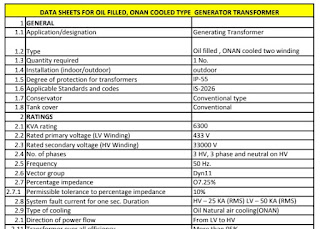13. Transformer Sizing

1. What is a Transformer
2. What are the different types of transformers
3. Different parts of transformer
4. Data sheet of transformer
5. Sizing of transformer
6. Tests for Transformer
7. Maintenance of transformer
8. Protection for Transformer
9. Misc content

1. What is a Transformer

• Transformer is a
• Static device
• Which transfers electric power from one circuit to other circuit
• With change in voltage level

• Working Principle
• Mutual Induction

• Picture of Transformer in a system

• 2. Different Types of Transformer

• On the base of Application
• Power Transformer
• Distribution transformer
• On the base of cooling
• Oil type
• Dry type
• On the base of Construction
• Core type
• Shell type
• On the base of number of windings
• Two winding
• Three winding
• Single winding

3. Different Parts of Transformer

4. Data sheet of TransformerLink

There will be 100 parameters in data sheet and design Engineer must know the importance of each parameter

5.  Sizing of Transformer

Transformer Sizing means, finding out the KVA rating of transformer
KVA rating means, the amount of power it can carry through it.
(* Transformer neither generates power, not converts to other form, it just carry)

To calculate the KVA rating, we should know the characteristic of load, means

• What is the connected load
• What is the peak load
• To arrive peak load, we will use demand factor and diversity factors
• while arriving peak load, we should consider the load characteristic i.e
• Once peak load is arrived, add 20% design margin and finalize the size in KVA
• Now, we have calculated the size of transformer
• But, here two conditions to be satisfied
• Voltage regulation during normal condition
• should be less than 5%
• Voltage regulation during starting of the motor
• Should be less than 10%
• If both conditions are satisfied, then we can finalise the KVA, other wise increase the transformer capacity to the next available standard size and check the voltage regulation condition. Once it is satisfied, we can conclude the size of the transformer in KVA

6. Tests for Transformer
• Routine tests
• Measurement of winding resistance
• Measurement of insulation resistance
• Seperate source voltage withstand test (High Voltage tests on HV & LV)
• Induced Over voltage Withstand test (DVDF test)- This test checks the inter turn insulation
• Measurement of voltage ratio
• Measurement of NO LOAD LOSS & current.
• Measurement of LOAD LOSS & IMPEDENCE.(EFFICIENCY & REGULATION)
• Vector Group Verification test
• Type tests
• All routine tests
• Lightning impulse test
• Temperature rise test
• Noise level test
• Special tests
• Partial discharge test
• Vibration test
• Tan-delta test
• Site tests
• IR value of transformer and cables
• Winding Resistance
• Transformer Turns Ratio
• Polarity Test
• Magnetizing Current
• Vector Group
• Magnetic Balance
• Bushing & Winding Tan Delta (HV )
• Protective relay testing
• Transformer oil testing
• Hipot test
7. What is maintenance of Transformer

Power transformers need regular periodic maintenance

• Operating conditions
• Ambient temperature
• Oil temperature
• Voltages
• Currents
• Checking tap postions
• General conditions
• Unusual noise
• Check oil level
• Check bushings
• Transformer
• Bushing insulators fine crack
• External electric connections and its tightness
• Breather condition
• check and test operation
• Alarms
• Relays
• Wiring
• Insulation resistance
• Ground connections and recording of earth resistance at electrodes
• Oil test
• Tightness of all cover bolts
• Check piping of oil and water
• Plugs and valves
• Heat exchangers
• Oil pumps
• Cooling fans
• Lightning arrestors and Spark gaps
• Overhaul

7. Protection of Transformer

• Over current protections
• Earth fault protection
• Differential Protection
• Restricted earth fault protections
• Gas detection
• Over voltage Protection
• Under frequency protection

8. Useful Content

• Why Transformer is rated in KVA, not in KW ?
• What is vector group in transformer ?
• What is % impedance of Transformer ?
• Meaning of Each parameter of Data sheet ?
• Maintenance in detail
• Tests in Detail
• Protection in detail

9. Why Transformer is rated in KVA, not in KW ?

• Loads will consume both active (KW) and reactive power (KVAR)
• Transformer have to carry both active and reactive power
• when we are calculating size of the transformer, we should consider both the consumption's Active and Reactive of the load
• So it is appropriate to mention KVA (which is combination of both active and reactive) rather KW alone.
10. What is vector group of transformer ?

The conditions to be met for connecting two transformers in parallel is

• Same Voltage ratio
• Same phase angle shift (vector group)
• Same polarity
• Same phase sequence
• Same frequency
• Same % impedance
• Same X/R ratio
• Same KVA rating
Now, What is phase angle shift:

More details will come soon.....

11.What is % impedance in transformer

Transformer will have winding inside, winding is nothing but conductor is wounded around core.
When aluminium or copper conductor is wounded, there will resistance and reactance.
Resistance plus reactance is nothing but imepdance of transformer.

But this impedance will be primary side and secondary side.